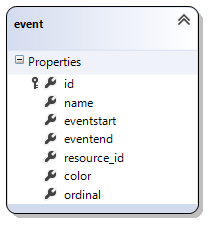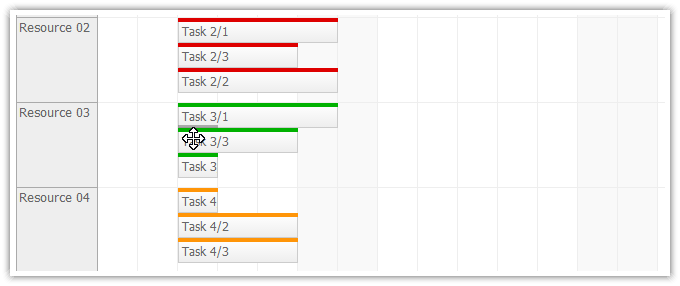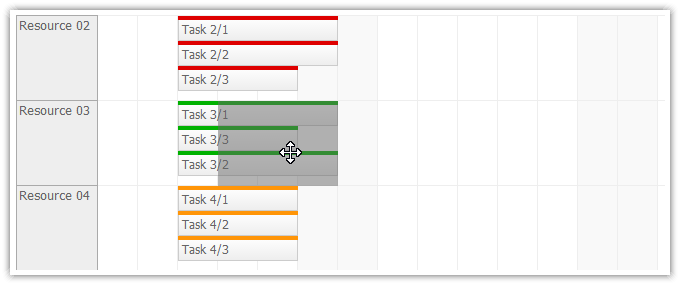## Features

This tutorial shows how to enable custom event sorting inside ASP.NET scheduler time cells. The default sorting corresponds "start asc, end desc".

• Custom event sorting using a dedicated database fields ("ordinal").
• The event order can be updated by drag and drop to a new position.
• Visual Studio 2012 Solution
• C# and VB source code
• The sample project includes a trial version of DayPilot Pro for ASP.NET WebForms package.

For an introduction to using the Scheduler control please see the ASP.NET Scheduler Tutorial.

Licensed for testing and evaluation purposes. You can use the source code of the tutorial if you are a licensed user of DayPilot Pro for ASP.NET WebForms. Buy a license.

## Sorting Scheduler EventsThis tutorial uses an SQL Server database with event stored in [event] table:

```CREATE TABLE [dbo].[event] (
[id]          INT          IDENTITY (1, 1) NOT NULL,
[name]        VARCHAR (50) NULL,
[eventstart]  DATETIME     NOT NULL,
[eventend]    DATETIME     NOT NULL,
[resource_id] INT          NULL,
[color]       VARCHAR (50) NULL,
[ordinal]     INT          NULL,
CONSTRAINT [PK_event] PRIMARY KEY CLUSTERED ([id] ASC)
);
```

The [ordinal] field stores the event order.

We will load the events and set the field that will be used for sorting concurrent events (EventSortExpression property).

C#

```private void SetDataSourceAndBind(DateTime start, DateTime end)
{
Scheduler.DataSource = GetData(start, end);

Scheduler.DataStartField = "eventstart";
Scheduler.DataEndField = "eventend";
Scheduler.DataIdField = "id";
Scheduler.DataTextField = "name";
Scheduler.DataResourceField = "resource_id";

Scheduler.EventSortExpression = "ordinal";

Scheduler.DataBind();
}```

VB

```Private Sub SetDataSourceAndBind(ByVal start As Date, ByVal [end] As Date)
Scheduler.DataSource = GetData(start, [end])

Scheduler.DataStartField = "eventstart"
Scheduler.DataEndField = "eventend"
Scheduler.DataIdField = "id"
Scheduler.DataTextField = "name"
Scheduler.DataResourceField = "resource_id"

Scheduler.EventSortExpression = "ordinal"
Scheduler.DataBind()

End Sub```

## Drag and Drop Moving to a Specific Position in a Day CellBy default, the scheduler events can by moved to a selected date and resource:We will enable moving to a specific position using EventMoveToPosition property:

```<DayPilot:DayPilotScheduler
ID="Scheduler"
runat="server"
...
EventMoveToPosition="true"
/>
```

## Updating the Event Position

The scheduler fires the EventMove server-side event after the event is dropped at the new place.

It's necessary to recalculate the sorting field after an event is moved to a new position (in the EventMove handler).

C#

```protected void Scheduler_EventMove(object sender, EventMoveEventArgs e)
{
if (e.OldResource != e.NewResource)
{
UpdateOldRow(e);
}

UpdateNewRow(e);

SetDataSourceAndBind(Scheduler.StartDate, Scheduler.EndDate);
Scheduler.UpdateWithMessage("Moved.");

}
```

VB

```Protected Sub Scheduler_EventMove(ByVal sender As Object, ByVal e As EventMoveEventArgs)
If e.OldResource <> e.NewResource Then
UpdateOldRow(e)
End If

UpdateNewRow(e)

SetDataSourceAndBind(Scheduler.StartDate, Scheduler.EndDate)
Scheduler.UpdateWithMessage("Moved.")

End Sub```

The UpdateOldRow() method recalculates the ordinals for the original row. It's not strictly necessary but it ensures that the sort order field values are continuous.

C#

```private void UpdateOldRow(EventMoveEventArgs e)
{
int thisId = Convert.ToInt32(e.Value);

// get a block of concurrent events at the old event position
DataTable concurrent = GetConcurrent(e.OldStart, e.OldEnd, Convert.ToInt32(e.OldResource));

// reset the ordinals
int i = 0;
foreach (DataRow row in concurrent.Rows)
{
int id = (int)row["id"];
if (id == thisId)
{
continue;
}
DbUpdateEventOrdinal(id, i);
i++;
}

}
```

VB

```Private Sub UpdateOldRow(ByVal e As EventMoveEventArgs)
Dim thisId As Integer = Convert.ToInt32(e.Value)

' get a block of concurrent events at the old event position
Dim concurrent As DataTable = GetConcurrent(e.OldStart, e.OldEnd, Convert.ToInt32(e.OldResource))

' reset the ordinals
Dim i As Integer = 0
For Each row As DataRow In concurrent.Rows
Dim id_Renamed As Integer = DirectCast(row("id"), Integer)
If id_Renamed = thisId Then
Continue For
End If
DbUpdateEventOrdinal(id_Renamed, i)
i += 1
Next row

End Sub
```

The UpdateNewRow() method updates the moved event and resets the ordinal field in the target row.

C#

```private void UpdateNewRow(EventMoveEventArgs e)
{
int thisId = Convert.ToInt32(e.Value);

// get a list of events concurrent with the new position
DataTable concurrent = GetConcurrent(e.NewStart, e.NewEnd, Convert.ToInt32(e.NewResource));

// check if the event is moved within the same block of concurrent events
int oldPosition = Position(thisId, concurrent);

// if the event was moved to a higher position inside the same block, adjust the position
int newPosition = e.Position;
if (oldPosition > -1 && oldPosition < newPosition)
{
newPosition -= 1;
}

// set the new resource, start, end, and position
DbUpdateEvent(thisId, e.NewResource, e.NewStart, e.NewEnd, e.Text, newPosition);

// reset the ordinals for all events in the block
int i = 0;
foreach (DataRow row in concurrent.Rows)
{
int id = (int)row["id"];
if (id == thisId)
{
continue;
}
if (i == newPosition)
{
i++;
}

DbUpdateEventOrdinal(id, i);
i++;
}
}

private int Position(int id, DataTable table)
{
for (int i = 0; i < table.Rows.Count; i++)
{
if ((int) table.Rows[i]["id"] == id)
{
return i;
}
}
return -1;
}
```

VB

```Private Sub UpdateNewRow(ByVal e As EventMoveEventArgs)
Dim thisId As Integer = Convert.ToInt32(e.Value)

' get a list of events concurrent with the new position
Dim concurrent As DataTable = GetConcurrent(e.NewStart, e.NewEnd, Convert.ToInt32(e.NewResource))

' check if the event is moved within the same block of concurrent events
Dim oldPosition As Integer = Position(thisId, concurrent)

' if the event was moved to a higher position inside the same block, adjust the position
Dim newPosition As Integer = e.Position
If oldPosition > -1 AndAlso oldPosition < newPosition Then
newPosition -= 1
End If

' set the new resource, start, end, and position
DbUpdateEvent(thisId, e.NewResource, e.NewStart, e.NewEnd, e.Text, newPosition)

' reset the ordinals for all events in the block
Dim i As Integer = 0
For Each row As DataRow In concurrent.Rows
Dim id_Renamed As Integer = DirectCast(row("id"), Integer)
If id_Renamed = thisId Then
Continue For
End If
If i = newPosition Then
i += 1
End If

DbUpdateEventOrdinal(id_Renamed, i)
i += 1
Next row

End Sub

Private Function Position(ByVal id As Integer, ByVal table As DataTable) As Integer
For i As Integer = 0 To table.Rows.Count - 1
If CInt(Math.Truncate(table.Rows(i)("id"))) = id Then
Return i
End If
Next i
Return -1
End Function
```